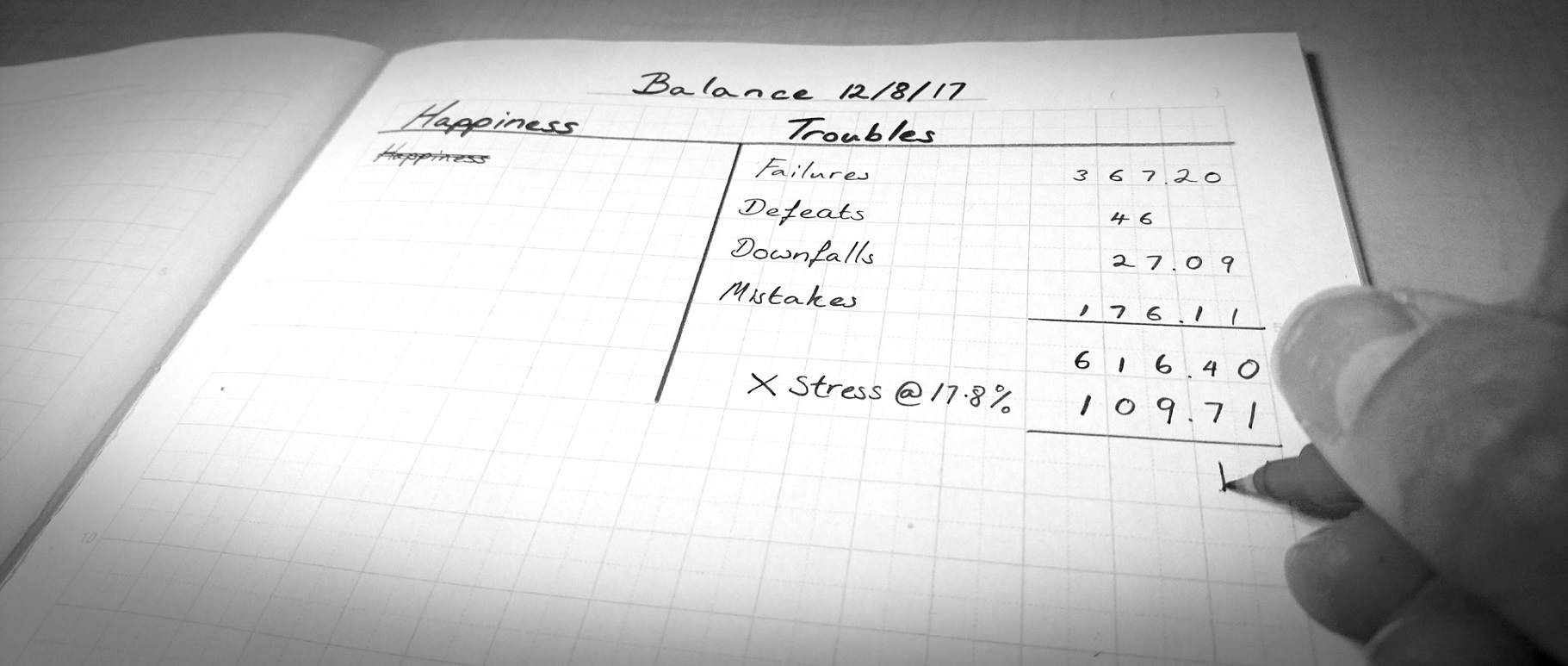## How to Analyse a Balance Sheet of an Organisation?

A balance sheet is a financial statement which states the financial position of an organisation on a particular date. It gives a quick snapshot of an organisation’s financial health. It comprises of two main elements i.e. Assets and Liabilities. A balance sheet displays the total assets and liabilities of an organisation along with the detailed bifurcation of the two. The assets are classified into two main head that is fixed asset (non-current asset) and current asset while liabilities is bifurcated into three main head which are Shareholder’s fund, long-term borrowing and current liability. One should carefully analyse an organisation’s balance sheet before it takes any stake in the organisation. Let’s look at the steps in which one could easily analyse and get useful insights from a balance sheet.

• First look at the assets side of a balance sheet. It comprises of two main head which is Current asset and Non- current asset. Fixed assets (non-current asset) are those assets that are used for more than one financial period by a firm while current assets are those assets that are consumed within a financial year. Look at the total of fixed assets and current asset. You can use these totals to calculate ratios such as return on asset (ROA), working capital ratio, proprietary ratio etc.

• Next, you look at the liabilities side of the balance sheet. You will find the total of the shareholder’s fund, long term borrowing (non-current liabilities) and current liabilities. If the total of shareholder’s fund is more than long term borrowing, then it means that the firm is less risky and are dependent more on the owner’s fund which is supposed to be a good practice. Then, look at the reserves and surplus of the firm which you can found under the head Shareholder’s fund. Higher the value of reserves and surplus stronger is the firm financial health.

• Compare the value of current assets with current liabilities. The firm is in a good position If the value of the current assets is more than the current liabilities. You can calculate the working capital of the firm by finding the difference between the value of current assets and current liabilities.

• You can evaluate the liquidity position of a firm through ratios such as current ratio and quick ratio which are calculated by dividing current assets with current liabilities and quick assets with current liabilities respectively. The ideal current ratio is 2:1 while the ideal quick ratio is 1:1.

• You can determine the solvency position of a firm by calculating solvency ratios such as debt-equity ratio, proprietary ratio etc. The debt-equity ratio is calculated by dividing the outsider’s fund with the shareholder’s fund while the proprietary ratio is measured by dividing the shareholder’s fund with the total asset. The ideal debt-equity ratio is 2:1. While the higher the proprietary ratio better is the firm’s solvency position.

• You can also determine the profitability of the firm through ratios such as return on equity (ROE) and return on capital employed (ROCE). A higher ratio implies a high firm’s profitability.

Apexdroid is cloud accounting software that auto generates the financial statements of an organisation. It is one of the best ERP systems for small business.

Apexdroid ERP integrates all core process that needed to grow a company:- Accounting, Finance, Payroll, Manufacturing, SCM, Procurement, CRM and others.Try Now: https://www.apexdroid.com